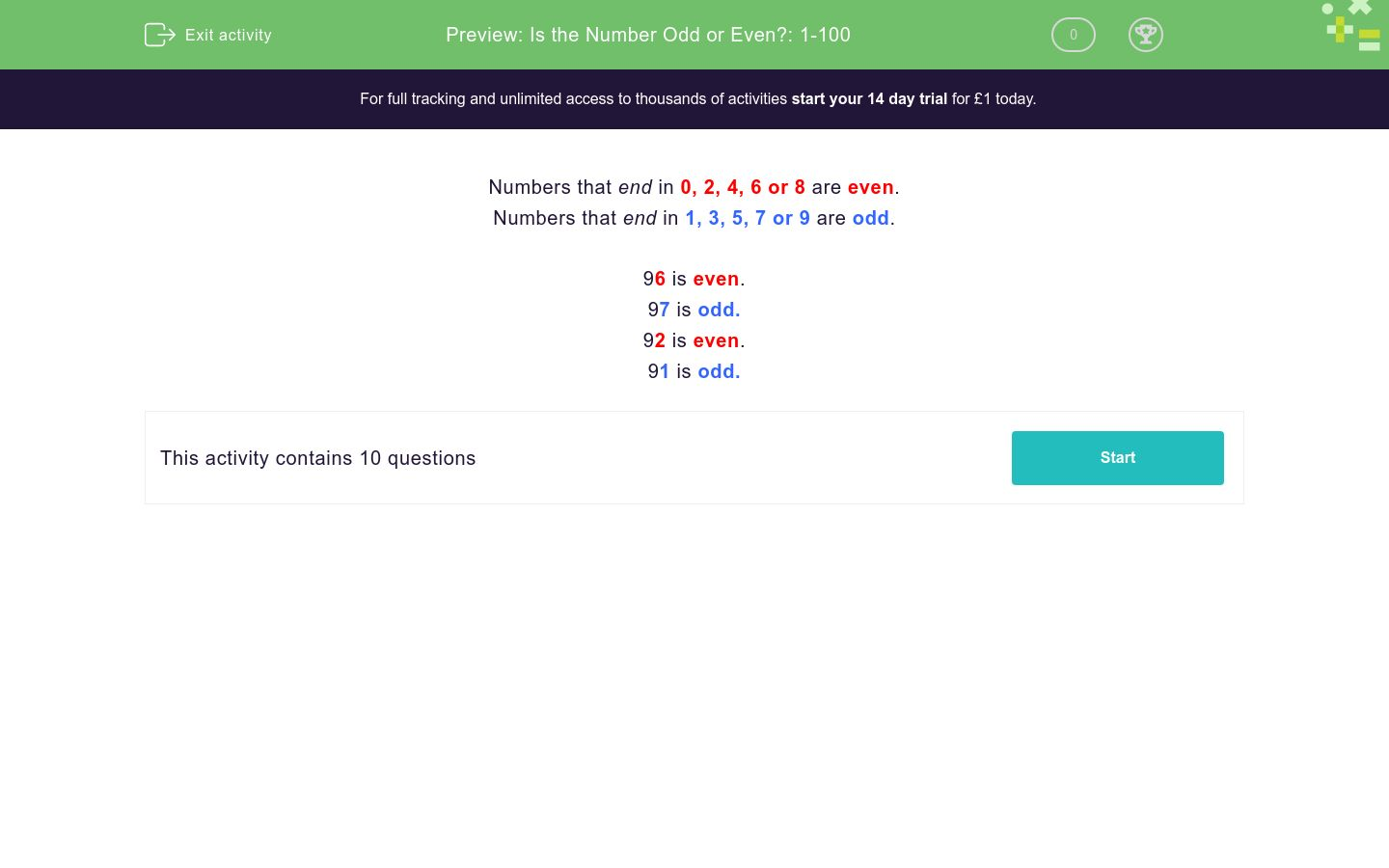# Is the Number Odd or Even?: 1-100

In this worksheet, students identify whether the given numbers are odd or even.Key stage:  KS 1

Curriculum topic:   Number: Number and Place Value

Curriculum subtopic:   Solve Place Value Problems to 100

Difficulty level:### QUESTION 1 of 10

Numbers that end in 0, 2, 4, 6 or 8 are even.

Numbers that end in 1, 3, 5, 7 or 9 are odd.

96 is even.

97 is odd.

92 is even.

91 is odd.

Is this number odd or even?

28

odd

even

Is this number odd or even?

56

odd

even

Is this number odd or even?

39

odd

even

Is this number odd or even?

99

odd

even

Is this number odd or even?

66

odd

even

Is this number odd or even?

34

odd

even

Is this number odd or even?

35

odd

even

Is this number odd or even?

62

odd

even

Is this number odd or even?

61

odd

even

Is this number odd or even?

57

odd

even

• Question 1

Is this number odd or even?

28

even
EDDIE SAYS
It ends in 8 which is even.
• Question 2

Is this number odd or even?

56

even
EDDIE SAYS
It ends in 6 which is even.
• Question 3

Is this number odd or even?

39

odd
EDDIE SAYS
It ends in 9 which is odd.
• Question 4

Is this number odd or even?

99

odd
EDDIE SAYS
It ends in 9 which is odd.
• Question 5

Is this number odd or even?

66

even
EDDIE SAYS
It ends in 6 which is even.
• Question 6

Is this number odd or even?

34

even
EDDIE SAYS
It ends in 4 which is even.
• Question 7

Is this number odd or even?

35

odd
EDDIE SAYS
It ends in 5 which is odd.
• Question 8

Is this number odd or even?

62

even
EDDIE SAYS
It ends in 2 which is even.
• Question 9

Is this number odd or even?

61

odd
EDDIE SAYS
It ends in 1 which is odd.
• Question 10

Is this number odd or even?

57

odd
EDDIE SAYS
It ends in 7 which is odd.
---- OR ----

Sign up for a £1 trial so you can track and measure your child's progress on this activity.

### What is EdPlace?

We're your National Curriculum aligned online education content provider helping each child succeed in English, maths and science from year 1 to GCSE. With an EdPlace account you’ll be able to track and measure progress, helping each child achieve their best. We build confidence and attainment by personalising each child’s learning at a level that suits them.

Get started